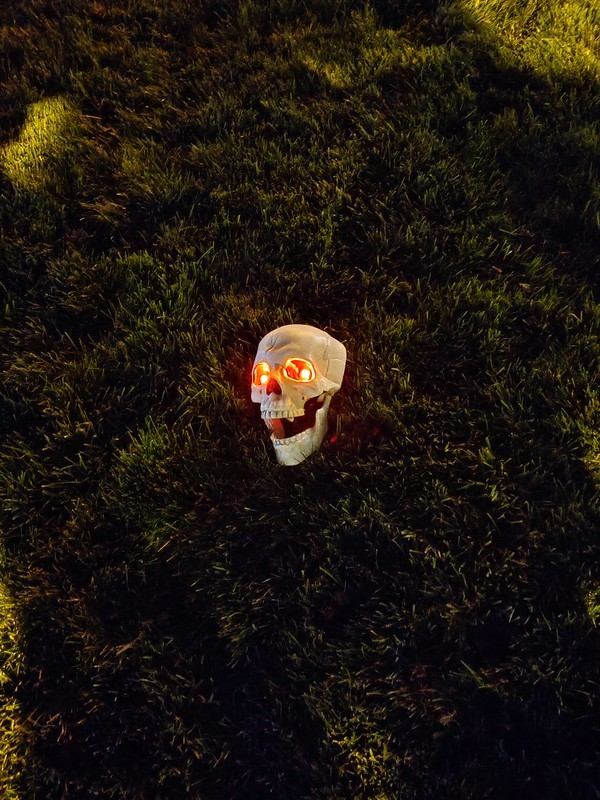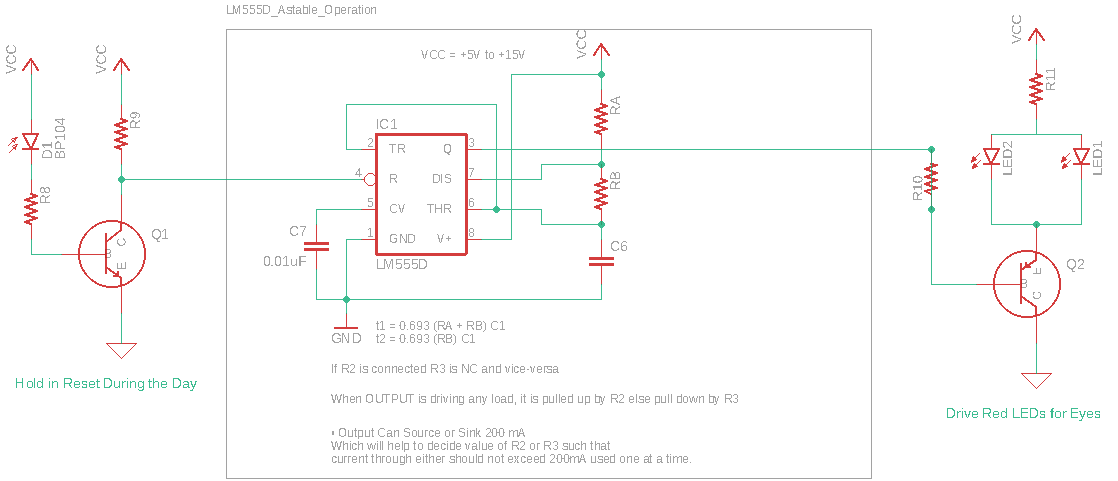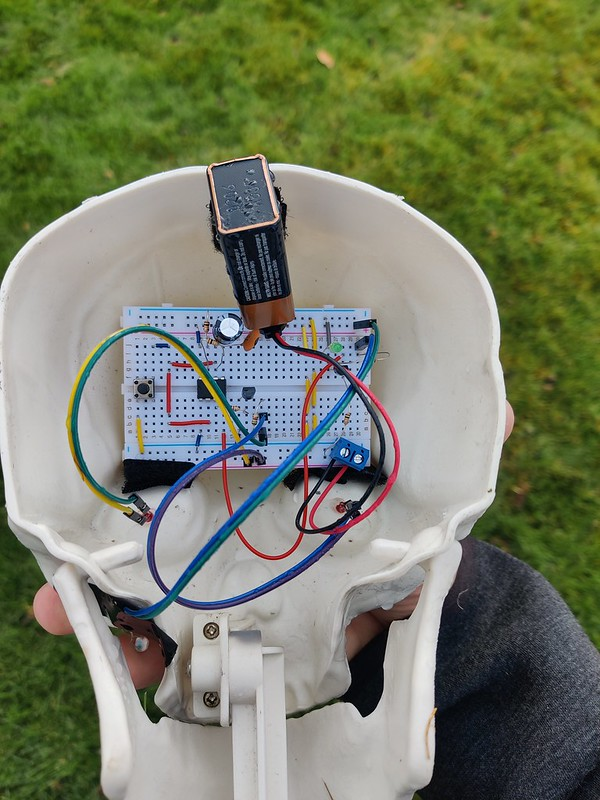## Lloyd Rochester's Geek Blog

For Halloween I attempted to do a little electronics with my young daughters to make a scary Halloween Skull with Blinking Red Eyes.# Circuit Functions

The circuit is easy to build and consists of 4 parts.

1. Photo Diode that triggers the circuit to be activated only at night
2. LM555 Timer to blink the Red LEDs for the Skull’s eyes
3. Transistors to Drive the diodes
4. A +9V Battery for Power

# Circuit

Here is the circuit we created. From left to right you can see the Photo Diode, the LM555 in astable mode, and the NPN Transistor powering the two red LEDs.The tricky part for me was to make the Photo Diode circuit trigger when it’s dark - inverse logic. As well as getting the power low so the +9V Battery will last multiple nights. More on that later.

# Assembly

I used Velcro to hold the circuit and battery into the Skull. I drilled holes for the eyes and used hot glue to keep them in. I did a little soldering so the LEDs would stay in. You can see to the lower left - very small - the Photo Diode which needs to face toward the Sun.# Bill of Materials

Here are the values I used. Change the values for RA, RB and C6 for the red LEDs to blink at different times.

Component Value Description
D1 Photo Diode
R8 10k Resistor to Base
R9 10k Resistor to Collector
Q1 BC547B NPN BJT
LM555D LM555 Astable Mode
R10 10k Not 100% sure on this
LED1 Standard 20mA LED
LED2 Standard 20mA LED
R11 1.5k Drives power consumption
Q2 BC547B Drive to LEDs

We have the following Duty Cycle for on/off on the LM555 Timer.

$t_{HIGH} = 0.693 (R_A + R_B) C6 = 0.693 * 20k\Omega * 1000\mu F = 13.86s$

and

$t_{LOW} = 0.683 R_B C = 0.693 * 10k\Omega * 1000\mu F = 6.93s$

where the Duty cycle $D$ is:

$D = \frac{R_B}{R_A+2R_B} = \frac{20k\Omega}{30k\Omega} = 66 \%$

# Power

Bare with me this is some RUSTY math!

Since I’m using a +9V battery I estimate it has roughtly 500mAH of juice.

First the current through Q2’s collector for two LED eyes is the +9V battery less the LED drop through the $1.5k\Omega$ resistor:

For the LED Driver we have appoximately:

$I_{B} = \frac{9V-0.7V}{10k \Omega } = 0.83mA$

$I_{C} = \frac{9V-0.7V}{1.5k \Omega} = 5.5mA$

For the Photo Diode in the Daylight we have: $I_{B} = 2*0.83mA = 1.66mA$

For the LM555 we can take appoximately 1mA during the night, otherwise it’s in reset. Thus, when the circuit is on it takes.

$I_{ON} = 0.83+5.5+1 = 7.33mA$

and when off let’s say it’s just using the power to hold the LM555 in reset for the photo diode:

$I_{OFF} = 1.66mA$

So for a 24 hour day we’d have 12 hours using $1.66mA$ and 12 hours when the circuit is on. Of those 12 hours only 66% use the full power for about 59mA of power total. The other off time we use 6.6mA total.

$I_{DAY} = 1.66mA12H(1+0.33) + 7.33mA12H*0.66 = \frac{85mAH}{day}$

So we can then take:

$\frac{500mAH}{85maH} = 5.88 Days$

I’d say this is on the upper range. We’ll see what it really is ….